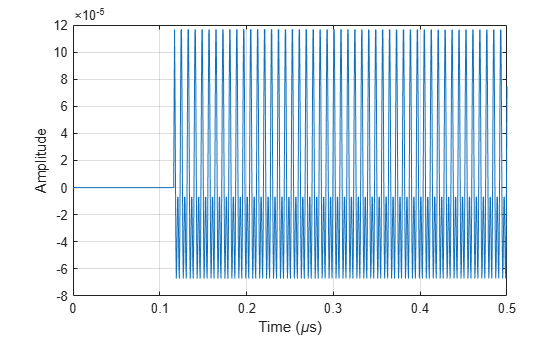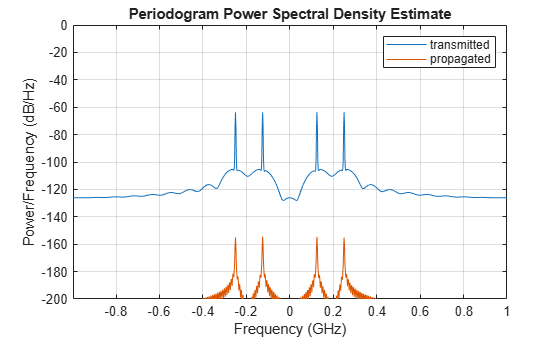# phased.WidebandLOSChannel

Wideband LOS propagation channel

## Description

The phased.WidebandLOSChannel models the propagation of narrowband electromagnetic signals through a line-of-sight (LOS) channel from a source to a destination. In an LOS channel, propagation paths are straight lines from point to point. The propagation model in the LOS channel includes free-space attenuation in addition to attenuation due to atmospheric gases, rain, fog, and clouds. You can use phased.WidebandLOSChannel to model the propagation of signals between multiple points simultaneously. The System object™ works for all frequencies.

While the attenuation models for atmospheric gases and rain are valid for electromagnetic signals in the frequency range 1–1000 GHz only, the attenuation model for fog and clouds is valid for 10–1000 GHz. Outside these frequency ranges, the System object uses the nearest valid value.

The phased.WidebandLOSChannel System object applies range-dependent time delays to the signals, as well as gains or losses. When either the source or destination is moving, the System object applies Doppler shifts.

Like the phased.WidebandFreeSpace System object, the phased.WidebandLOSChannel System object supports two-way propagation.

To compute the propagation delay for specified source and receiver points:

1. Define and set up your Wideband LOS channel using the Construction procedure. You can set the System object properties during construction or leave them at their default values.

2. Call the step method to compute the propagated signal using the properties of the phased.WidebandLOSChannel System object. You can change tunable properties before or after any call to the step method.

Note

Starting in R2016b, instead of using the step method to perform the operation defined by the System object, you can call the object with arguments, as if it were a function. For example, y = step(obj,x) and y = obj(x) perform equivalent operations.

## Construction

sWBLOS = phased.WidebandLOSChannel creates a Wideband LOS attenuating propagation channel System object, sWBLOS.

sWBLOS = phased.WidebandLOSChannel(Name,Value) creates a System object, sWBLOS, with each specified property Name set to the specified Value. You can specify additional name and value pair arguments in any order as (Name1,Value1,...,NameN,ValueN).

## Properties

expand all

Signal propagation speed, specified as a positive scalar. Units are in meters per second. The default propagation speed is the value returned by physconst('LightSpeed'). See physconst for more information.

Example: 3e8

Data Types: double

Operating frequency, specified as a positive scalar. Units are in Hz.

Example: 1e9

Data Types: double

Option to enable the atmospheric attenuation model, specified as a false or true. Set this property to true to add signal attenuation caused by atmospheric gases, rain, fog, or clouds. Set this property to false to ignore atmospheric effects in propagation.

Setting SpecifyAtmosphere to true, enables the Temperature, DryAirPressure, WaterVapourDensity, LiquidWaterDensity, and RainRate properties.

Data Types: logical

Ambient temperature, specified as a real-valued scalar. Units are in degrees Celsius.

Example: 20.0

#### Dependencies

To enable this property, set SpecifyAtmosphere to true.

Data Types: double

Atmospheric dry air pressure, specified as a positive real-valued scalar. Units are in pascals (Pa). The default value of this property corresponds to one standard atmosphere.

Example: 101.0e3

#### Dependencies

To enable this property, set SpecifyAtmosphere to true.

Data Types: double

Atmospheric water vapor density, specified as a positive real-valued scalar. Units are in g/m3.

Example: 7.4

#### Dependencies

To enable this property, set SpecifyAtmosphere to true.

Data Types: double

Liquid water density of fog or clouds, specified as a nonnegative real-valued scalar. Units are in g/m3. Typical values for liquid water density are 0.05 for medium fog and 0.5 for thick fog.

Example: 0.1

#### Dependencies

To enable this property, set SpecifyAtmosphere to true.

Data Types: double

Rainfall rate, specified as a nonnegative real-valued scalar. Units are in mm/hr. This property applies only when you set SpecifyAtmosphere to true.

Example: 10.0

Data Types: double

Enable two-way propagation, specified as a false or true. Set this property to true to perform round-trip propagation between the signal origin and destination specified in step. Set this property to false to perform only one-way propagation from the origin to the destination.

Example: true

Data Types: logical

Sample rate of signal, specified as a positive scalar. Units are in Hz. The System object uses this quantity to calculate the propagation delay in units of samples.

Example: 1e6

Data Types: double

Number of processing subbands, specified as a positive integer.

Example: 128

Data Types: double

Source of maximum one-way propagation distance, specified as 'Auto' or 'Property'. The maximum one-way propagation distance is used to allocate sufficient memory for signal delay computation. When you set this property to 'Auto', the System object automatically allocates memory. When you set this property to 'Property', you specify the maximum one-way propagation distance using the value of the MaximumDistance property.

Data Types: char

Maximum one-way propagation distance, specified as a positive real-valued scalar. Units are in meters. Any signal that propagates more than the maximum one-way distance is ignored. The maximum distance must be greater than or equal to the largest position-to-position distance.

Example: 5000

#### Dependencies

To enable this property, set the MaximumDistanceSource property to 'Property'.

Data Types: double

The source of the maximum number of samples of the input signal, specified as 'Auto' or 'Property'. When you set this property to 'Auto', the propagation model automatically allocates enough memory to buffer the input signal. When you set this property to 'Property', you specify the maximum number of samples in the input signal using the MaximumNumInputSamples property. Any input signal longer than that value is truncated.

To use this object with variable-size signals in a MATLAB® Function Block in Simulink®, set the MaximumNumInputSamplesSource property to 'Property' and set a value for the MaximumNumInputSamples property.

Example: 'Property'

#### Dependencies

To enable this property, set MaximumDistanceSource to 'Property'.

Data Types: char

Maximum number of input signal samples, specified as a positive integer. The input signal is the first argument of the step method, after the System object itself. The size of the input signal is the number of rows in the input matrix. Any input signal longer than this number is truncated. To process signals completely, ensure that this property value is greater than any maximum input signal length.

The waveform-generating System objects determine the maximum signal size:

• For any waveform, if the waveform OutputFormat property is set to 'Samples', the maximum signal length is the value specified in the NumSamples property.

• For pulse waveforms, if the OutputFormat is set to 'Pulses', the signal length is the product of the smallest pulse repetition frequency, the number of pulses, and the sample rate.

• For continuous waveforms, if the OutputFormat is set to 'Sweeps', the signal length is the product of the sweep time, the number of sweeps, and the sample rate.

Example: 2048

#### Dependencies

To enable this property, set MaximumNumInputSamplesSource to 'Property'.

Data Types: double

## Methods

 reset Reset states of System object step Propagate signal in Wideband LOS channel
Common to All System Objects
release

Allow System object property value changes

## Examples

collapse all

Propagate a wideband signal in a line-of-sight (LOS) channel from a radar at (0,0,0) meters to a target at (35,0,0) meters in medium fog. Set the fog liquid water density to 0.05 gm/m3. Assume rain is falling at 5 mm/hr. The signal carrier frequency is 20 GHz. The signal is a sum of four cw tones at 19.75, 19.875, 20.125, and 20.25 GHz. Set the signal duration to 0.5 μs and the sample rate to 2.0 GHz. Assume the radar is stationary and the target approaches the radar at 40 m/s. The atmospheric temperature is 12°C.

Set the signal parameters and create the transmitted signal.

c = physconst('LightSpeed'); fs = 2e9; freq = [-0.25,-.125,0.125,0.25]*1e9; fc = 20.0e9; dt = 1/fs; t = [0:dt:.5e-6]; sig = sum(exp(1i*2*pi*t.'*freq),2);

Specify the atmosphere parameters and create the phased.WidebandChannel System object™.

lwd = 0.05; rainrate = 5.0; temp = 12.0; loschannel = phased.WidebandLOSChannel('SampleRate',fs,'PropagationSpeed',c,... 'SpecifyAtmosphere',true,'OperatingFrequency',fc,'RainRate',rainrate,... 'LiquidWaterDensity',lwd,'Temperature',temp);

Specify the radar and target positions and velocities.

xradar = [0,0,0].'; vradar = [0,0,0].'; xtgt = [35,0,0].'; vtgt = [-40,0,0].';

Propagate the signal using the step method.

prop_sig = loschannel(sig,xradar,xtgt,vradar,vtgt);

Plot the propagated signal. For a target range of 35 m, the propagation delay is 0.11 μs as seen in the plot.

plot(t*1e6,real(prop_sig)) grid xlabel('Time ({\mu}s)') ylabel('Amplitude')Using the periodogram function with a Taylor window, plot the spectra of the original and propagated signals.

nfft = 1024; nsamp = size(sig,1); periodogram([sig prop_sig],taylorwin(nsamp),nfft,fs,'centered') ylim([-200 0]) legend('transmitted','propagated')expand all

## References

 Radiocommunication Sector of the International Telecommunication Union. Recommendation ITU-R P.676-10: Attenuation by atmospheric gases. 2013.

 Radiocommunication Sector of the International Telecommunication Union. Recommendation ITU-R P.840-6: Attenuation due to clouds and fog. 2013.

 Radiocommunication Sector of the International Telecommunication Union. Recommendation ITU-R P.838-3: Specific attenuation model for rain for use in prediction methods. 2005.

 Seybold, J. Introduction to RF Propagation. New York: Wiley & Sons, 2005.

 Skolnik, M. Introduction to Radar Systems, 3rd Ed. New York: McGraw-Hill, 2001.

## Version History

Introduced in R2016a